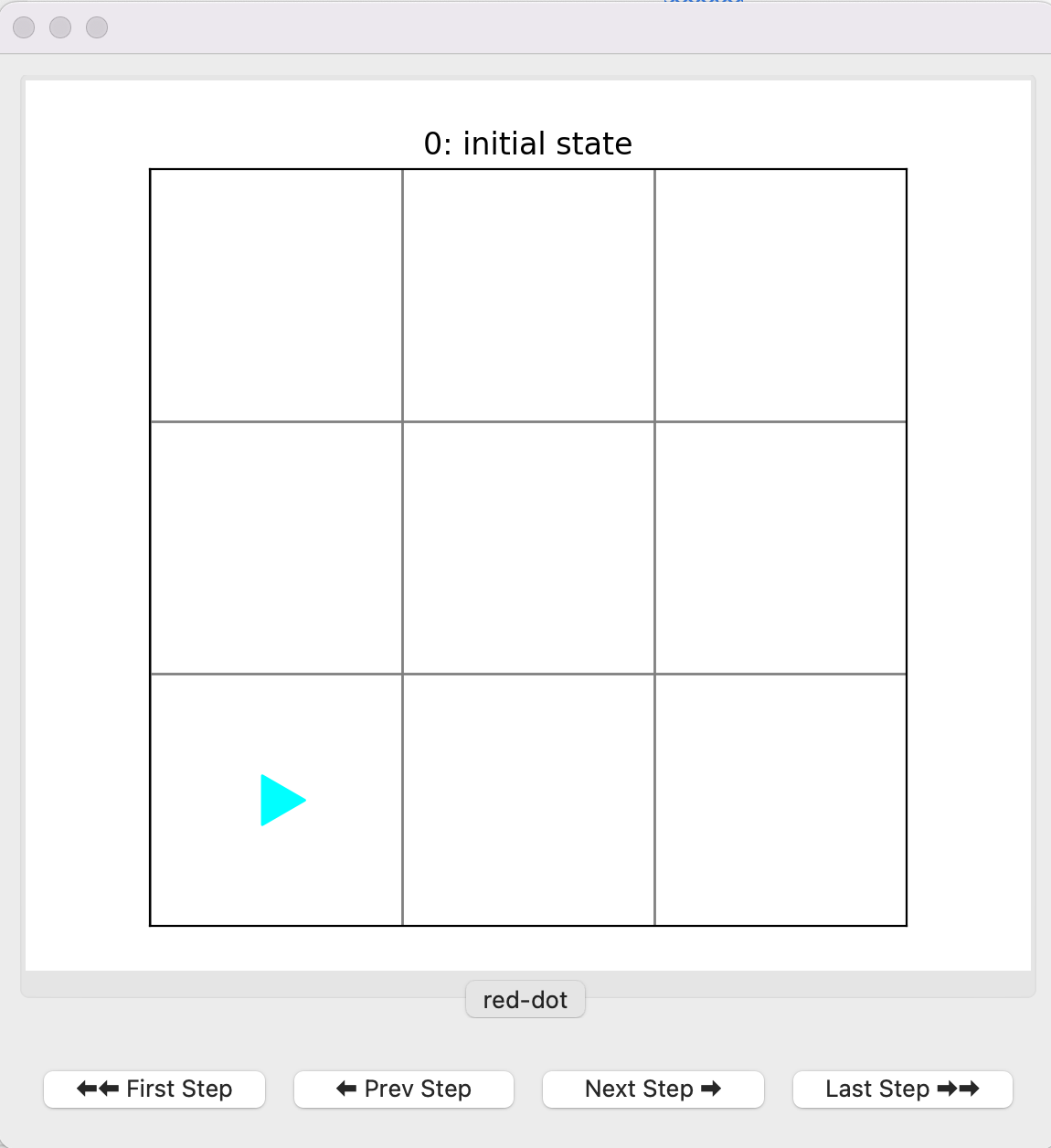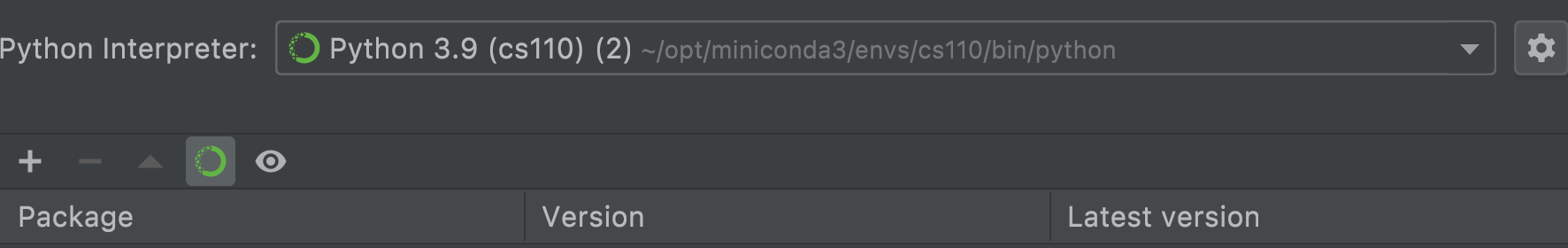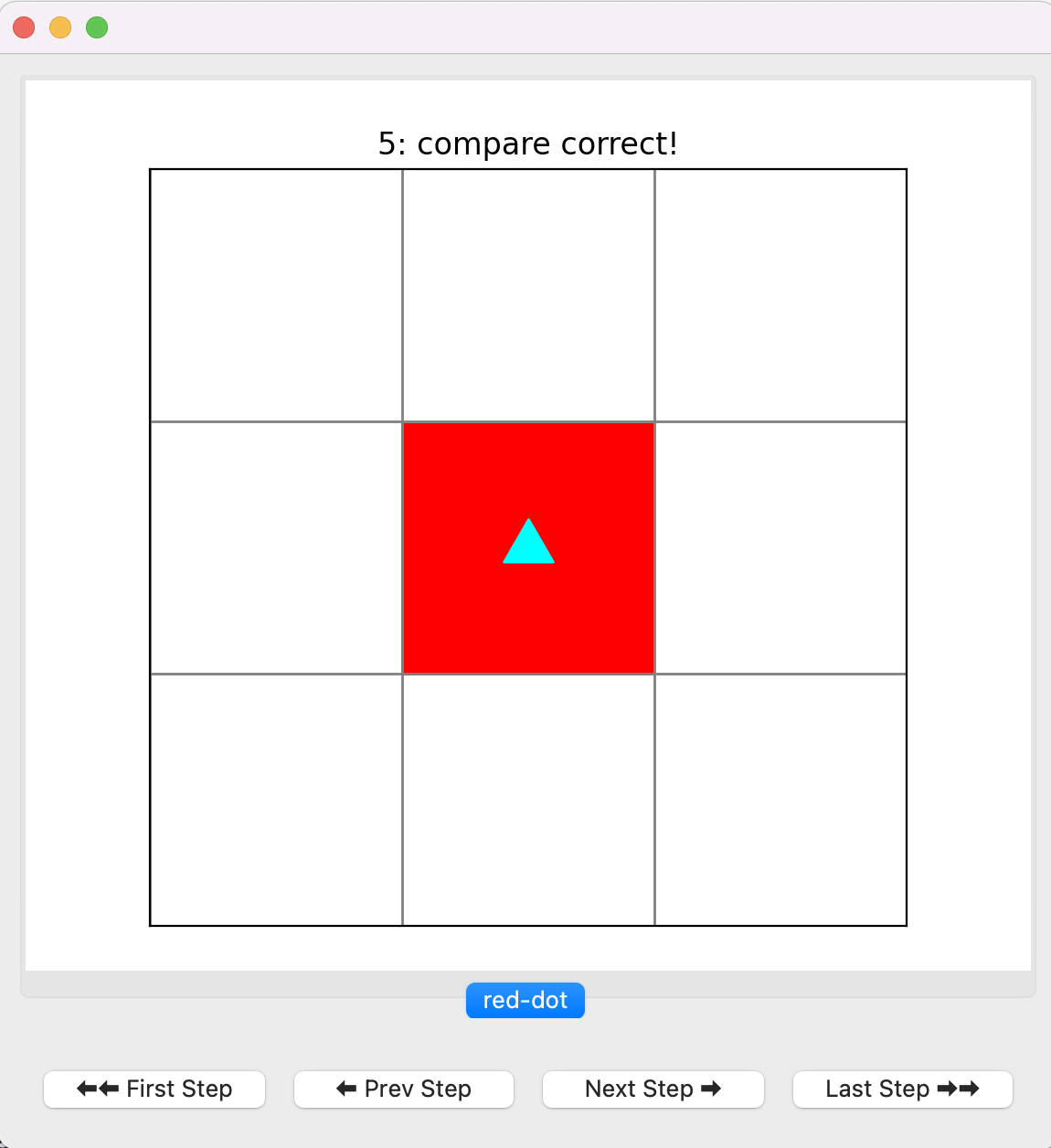Computer Science

# Bit Reference

The byubit library helps you learn to program by drawing pictures and solving puzzles. Bit works in a “world” of squares:You can think of Bit as a robot, in the shape of a triangle. Bit has a position and a direction. In the picture above, Bit is at the bottom left, facing right.

## Installing byubit

The most reliable way to install byubit is through PyCharm. Open the File->Settings (Windows) or File->Preferences (MacOS) menu and select Project. You should see a your Conda environment and a list of packages installed in that environment:Click the + and then type in byubit. Then click Install Package.

## Importing Bit

To use Bit, you always need to import it using the following:

from byubit import Bit

## Running Bit in a world.

You run bit in a world using:

@Bit.run(world-name)
def function_name(bit):
# write some code here

For example, you can run Bit on the red-dot world using a function called red_dot like this:

@Bit.run('red-dot')
def red_dot(bit):
# Implement me!
pass

This loads a world from a file called red-dot.start.txt. You can then write code in the function to control Bit.

## Moving Bit

You can move and turn Bit using the following functions:

• bit.move() — move forward one space
• bit.right() — turn right (without moving)
• bit.left() — turn left (without moving)

This example moves Bit a few spaces and turns:

bit.move()
bit.left()
bit.move()
bit.right()
bit.move()

## Painting colors

You can use the following functions to change the colors of squares:

• bit.paint(color) — paint the color of the current square; valid colors are ‘red’, ‘green’, and ‘blue’
• bit.get_color() — returns the color of the current square
• bit.erase() — erases the color of the current square

This example paints the current square red:

bit.paint('red')

This example gets the color of the current square and stores it in a variable:

current_color = bit.get_color()

## Checking colors

You can check the color of the current square:

• bit.is_red() — returns true if the current square is red
• bit.is_blue() — returns true if the current square is blue
• bit.is_green() — returns true if the current square is green

This example moves until the current square is not red:

while bit.is_red():
bit.move()

## Checking if a square is clear

Bit can’t move if the square in front of it is black, or if it reaches the end of its world. You can use the following methods to check squares near Bit:

• bit.front_clear() — checks if the square in front of Bit is clear (not black, not the end of the world)
• bit.right_clear() — checks if the square to the right of Bit is clear
• bit.left_clear() — checks if the square to the left of Bit is clear

This example moves Bit until it reaches the end of the world:

while bit.front_clear():
bit.move()

## Stepping

You can use the graphical Bit interface to step through every command that you give to Bit. By default, the interface runs your entire program and stops at the end:This shows that you are running the red-dot world and that your finish state matches the solution (“compare correct!”). You can use the Prev Step and Next Step buttons to go backwards and forward. You can use the First Step and Last Step buttons to go to the beginning or end.

## Snapshots

You can create a snapshot of the current bit state using:

• bit.snapshot(name) — creates a snapshot with the given name

Imagine you are trying to move Bit to the top right corner. This example creates a snapshot called halfway that stops after Bit should have reached the right side.

while bit.front_clear():
bit.move()
bit.snapshot('halfway')
bit.left()
while bit.front_clear():
bit.move()

This is helpful for debugging because you can go right to a particular point of your code instead of stepping through everything one command at a time.

## Summary

@Bit.run(world-name)
def function_name(bit):
# write some code here
• Moving
• bit.move() — move forward one space
• bit.right() — turn right (without moving)
• bit.left() — turn left (without moving)
• Painting
• bit.paint(color) — paint the color of the current square; valid colors are ‘red’, ‘green’, and ‘blue’
• bit.get_color() — returns the color of the current square
• bit.erase() — erases the color of the current square
• Checking Colors
• bit.is_red() — returns true if the current square is red
• bit.is_blue() — returns true if the current square is blue
• bit.is_green() — returns true if the current square is green
• Checking if a Square is Clear
• bit.front_clear() — checks if the square in front of Bit is clear (not black, not the end of the world)
• bit.right_clear() — checks if the square to the right of Bit is clear
• bit.left_clear() — checks if the square to the left of Bit is clear
• Snapshots
• bit.snapshot(name) — creates a snapshot with the given name